# Frank solutions for Class 9 Maths ICSE chapter 21 - Areas Theorems on Parallelograms [Latest edition]

#### Chapters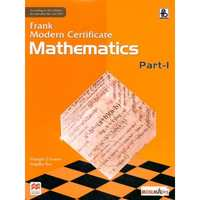## Chapter 21: Areas Theorems on Parallelograms

Exercise 21.1
Exercise 21.1 [Page 25]

### Frank solutions for Class 9 Maths ICSE Chapter 21 Areas Theorems on Parallelograms Exercise 21.1 [Page 25]

Exercise 21.1 | Q 1

ABCD is a parallelogram having an area of 60cm2. P is a point on CD. Calculate the area of ΔAPB.

Exercise 21.1 | Q 2

PQRS is a rectangle in which PQ = 12cm and PS = 8cm. Calculate the area of ΔPRS.

Exercise 21.1 | Q 3

In the figure, PT is parallel to SR. QTSR is a parallelogram and PQSR is a rectangle. If the area of ΔQTS is 60cm2, find:
(i) the area o || gm QTSR
(ii) the area of the rectangle PQRS
(iii) the area of the triangle PQS.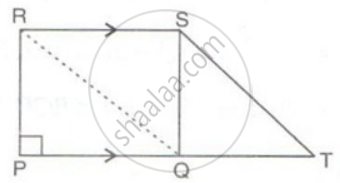Exercise 21.1 | Q 4

In the given figure area of ∥ gm PQRS is 30 cm2. Find the height of ∥ gm PQFE if PQ = 6 cm.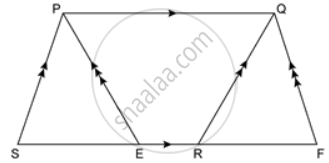Exercise 21.1 | Q 5

In the given figure, PQRS is a ∥ gm. A straight line through P cuts SR at point T and QR produced at N. Prove that area of triangle QTR is equal to the area of triangle STN.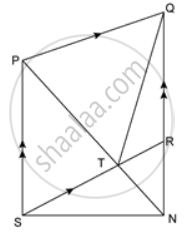Exercise 21.1 | Q 6

In the given figure, ST ∥ PR. Prove that: area of quadrilateral PQRS = area of ΔPQT.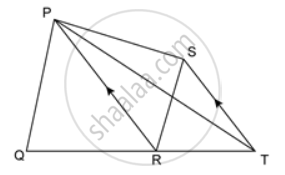Exercise 21.1 | Q 7

In the figure, ABCD is a parallelogram and APD is an equilateral triangle of side 80cm, Calculate the area of parallelogram ABCD.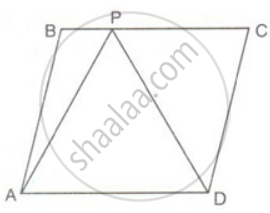Exercise 21.1 | Q 8

In the figure, if the area of ||gm PQRS is 84cm2; find the area of
(i) || gm PQMN
(ii) ΔPQS
(iii) ΔPQN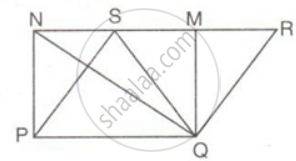Exercise 21.1 | Q 9

In the figure, PQR is a straight line. SQ is parallel to Tp. Prove that the quadrilateral PQST is equal in area to the ΔPSR.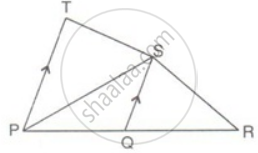Exercise 21.1 | Q 10

In the given figure, if AB ∥ DC ∥ FG and AE is a straight line. Also, AD ∥ FC. Prove that: area of ∥ gm ABCD = area of ∥ gm BFGE.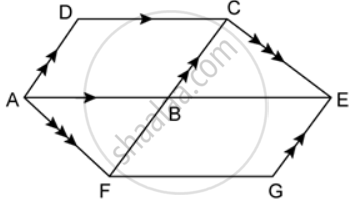Exercise 21.1 | Q 11

In the given figure, the perimeter of parallelogram PQRS is 42 cm. Find the lengths of PQ and PS.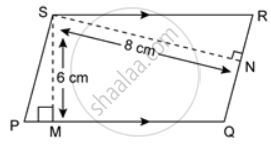Exercise 21.1 | Q 12

In the given figure, PT ∥ QR and QT ∥ RS. Show that: area of ΔPQR = area of ΔTQS.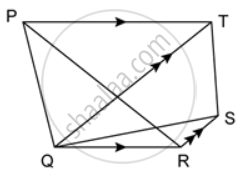*Question modified

Exercise 21.1 | Q 13

In the given figure, ΔPQR is right-angled at P. PABQ and QRST are squares on the side PQ and hypotenuse QR. If PN ⊥ TS, show that:
(a) ΔQRB ≅ ΔPQT
(b) Area of square PABQ = area of rectangle QTNM.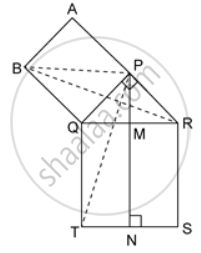Exercise 21.1 | Q 14

In the figure, AE = BE. Prove that the area of triangle ACE is equal in area to the parallelogram ABCD.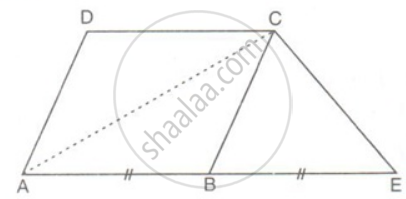Exercise 21.1 | Q 15

The diagonals of a parallelogram ABCD intersect at O. A line through O meets AB in P and CD in Q. Show that
(a) Area of APQD = (1)/(2) area of || gm ABCD

(b) Area of APQD = Area of BPQC

Exercise 21.1 | Q 16

Prove that the median of a triangle divides it into two triangles of equal area.

Exercise 21.1 | Q 17

AD is a median of a ΔABC.P is any point on AD. Show that the area of ΔABP is equal to the area of ΔACP.

Exercise 21.1 | Q 18

In the given figure AF = BF and DCBF is a parallelogram. If the area of ΔABC is 30 square units, find the area of the parallelogram DCBF.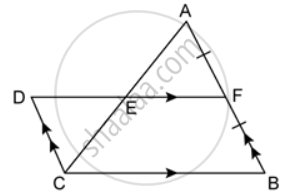Exercise 21.1 | Q 19

Prove that the diagonals of a parallelogram divide it into four triangles of equal area.

Exercise 21.1 | Q 20

The diagonals AC and BC of a quadrilateral ABCD intersect at O. Prove that if BO = OD, then areas of ΔABC an ΔADC area equal.

Exercise 21.1 | Q 21

Prove that the area of a rhombus is equal to half the rectangle contained by its diagonals.

Exercise 21.1 | Q 22

PQRS is a parallelogram and O is any point in its interior. Prove that: area(ΔPOQ) + area(ΔROS) - area(ΔQOR) + area(ΔSOP) = (1)/(2)area(|| gm PQRS)

Exercise 21.1 | Q 23

A quadrilateral ABCD is such that diagonals BD divides its area into two equal parts. Prove that BD bisects AC.

Exercise 21.1 | Q 24

In the given figure, BC ∥ DE.
(a) If area of ΔADC is 20 sq. units, find the area of ΔAEB.
(b) If the area of ΔBFD is 8 square units, find the area of ΔCEF

Exercise 21.1 | Q 26

ΔPQR and ΔSQR are on the same base QR with P and S on opposite sides of line QR, such that area of ΔPQR is equal to the area of ΔSQR. Show that QR bisects PS.

Exercise 21.1 | Q 27

If the medians of a ΔABBC intersect at G, show that ar(ΔAGB) = ar(ΔAGC) = ar(ΔBGC) = (1)/(3)"ar(ΔABC)".

Exercise 21.1 | Q 28

In ΔABC, the mid-points of AB, BC and AC are P, Q and R respectively. Prove that BQRP is a parallelogram and that its area is half of ΔABC.

Exercise 21.1 | Q 29

In the given figure, PQ ∥ SR ∥ MN, PS ∥ QM and SM ∥ PN. Prove that: ar. (SMNT) = ar. (PQRS).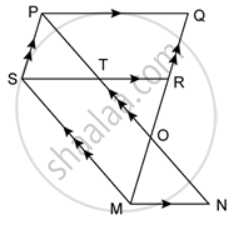Exercise 21.1 | Q 31

In ΔPQR, PS is a median. T is the mid-point of SR and M is the mid-point of PT. Prove that: ΔPMR = (1)/(8)Δ"PQR".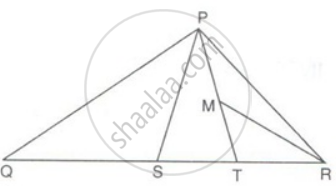Exercise 21.1 | Q 32

In the figure, ABCD is a parallelogram and CP is parallel to DB. Prove that: Area of OBPC = (3)/(4)"area of ABCD"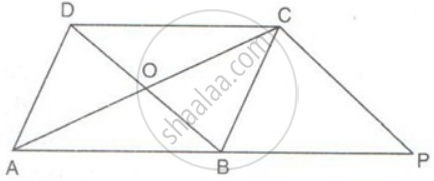Exercise 21.1 | Q 33

The medians QM and RN of ΔPQR intersect at O. Prove that: area of ΔROQ = area of quadrilateral PMON.

Exercise 21.1 | Q 34.1

In the given figure, ABC is a triangle and AD is the median.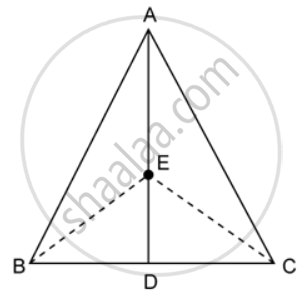If E is any point on the median AD. Show that: Area of ΔABE = Area of ΔACE.

Exercise 21.1 | Q 34.2

In the given figure, ABC is a triangle and AD is the median.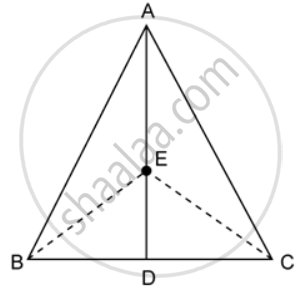If E is the midpoint of the median AD, prove that: Area of ΔABC = 4 × Area of ΔABE

Exercise 21.1 | Q 35

In a parallelogram PQRS, M and N are the midpoints of the sides PQ and PS respectively. If area of ΔPMN is 20 square units, find the area of the parallelogram PQRS.

Exercise 21.1 | Q 36

In a parallelogram PQRS, T is any point on the diagonal PR. If the area of ΔPTQ is 18 square units find the area of ΔPTS.

Exercise 21.1 | Q 37

ABCD is a quadrilateral in which diagonals AC and BD intersect at a point O. Prove that: areaΔAOD + areaΔBOC + areaΔABO + areaΔCDO.

Exercise 21.1 | Page 25

In the given figure, AB ∥ SQ ∥ DC and AD ∥ PR ∥ BC. If the area of quadrilateral ABCD is 24 square units, find the area of quadrilateral PQRS.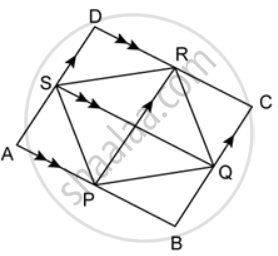## Chapter 21: Areas Theorems on Parallelograms

Exercise 21.1## Frank solutions for Class 9 Maths ICSE chapter 21 - Areas Theorems on Parallelograms

Frank solutions for Class 9 Maths ICSE chapter 21 (Areas Theorems on Parallelograms) include all questions with solution and detail explanation. This will clear students doubts about any question and improve application skills while preparing for board exams. The detailed, step-by-step solutions will help you understand the concepts better and clear your confusions, if any. Shaalaa.com has the CISCE Class 9 Maths ICSE solutions in a manner that help students grasp basic concepts better and faster.

Further, we at Shaalaa.com provide such solutions so that students can prepare for written exams. Frank textbook solutions can be a core help for self-study and acts as a perfect self-help guidance for students.

Concepts covered in Class 9 Maths ICSE chapter 21 Areas Theorems on Parallelograms are Introduction of Rectilinear Figures, Names of Polygons, Concept of Quadrilaterals - Sides, Adjacent Sides, Opposite Sides, Angle, Adjacent Angles and Opposite Angles, Diagonal Properties of Different Kinds of Parallelograms, Property: The Diagonals of a Rectangle Are of Equal Length., Property: The diagonals of a square are perpendicular bisectors of each other., Types of Quadrilaterals, Property: The Opposite Sides of a Parallelogram Are of Equal Length., Property: The Opposite Angles of a Parallelogram Are of Equal Measure., Property: The diagonals of a rhombus are perpendicular bisectors of one another..

Using Frank Class 9 solutions Areas Theorems on Parallelograms exercise by students are an easy way to prepare for the exams, as they involve solutions arranged chapter-wise also page wise. The questions involved in Frank Solutions are important questions that can be asked in the final exam. Maximum students of CISCE Class 9 prefer Frank Textbook Solutions to score more in exam.

Get the free view of chapter 21 Areas Theorems on Parallelograms Class 9 extra questions for Class 9 Maths ICSE and can use Shaalaa.com to keep it handy for your exam preparation